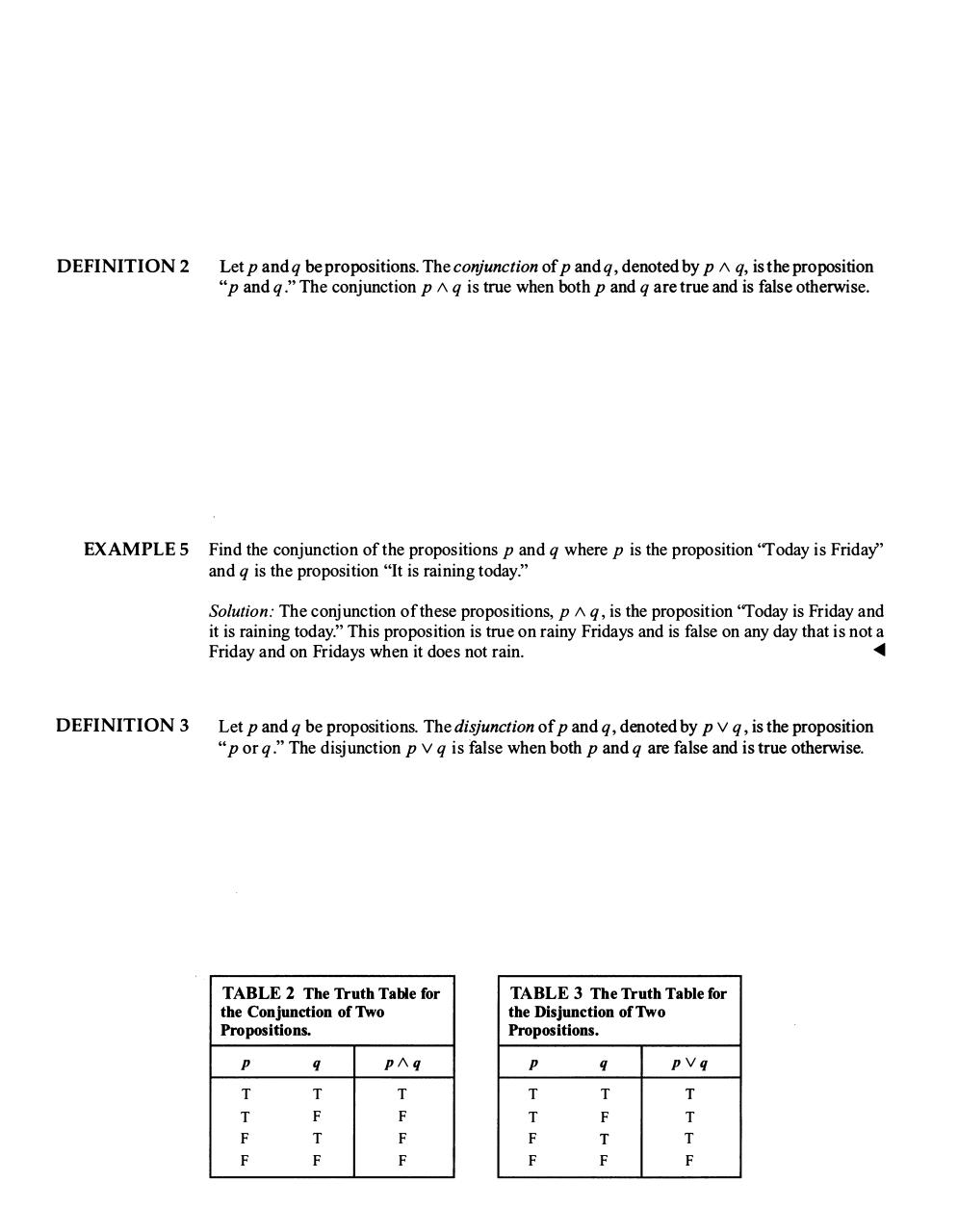# 1+&+2.pdfPage 1 2 34522

#### Text preview

DEFINITION 2

Let p and q be propositions. The conjunction of p and q , denoted by p /\ q, is the proposition
&quot;
&quot;
p and q . The conjunction p /\ q is true when both p and q are true and is false otherwise
.

EXAMPLE 5

Find the conjunction of the propositions p and q where p is the proposition &quot;Today is Friday&quot;
and q is the proposition &quot;It is raining today.&quot;

S olution: The conjunction of these propositions, p /\ q , is the proposition &quot;Today is Friday and

it is raining today.&quot; This proposition is true on rainy Fridays and is false on any day that is not a

Friday and on Fridays when it does not rain.

DEFINITION 3

Let p and q be propositions. The disjunction of p and q , denoted by p V q , is the proposition
&quot;
p or q . The disj unction p V q is false when both p and q are false and is true otherwise.

&quot;

TABLE 2 The Truth Table for
the Conjunction of Two
Propositions.
p

TABLE 3 The Truth Table for
the Disjunction of Two
Propositions.

q

p /\ q

p

q

pVq

T

T

T

T

T

T

T

F

F

T

F

T

F

T

F

F

F

F

F

F

T
F

T
F